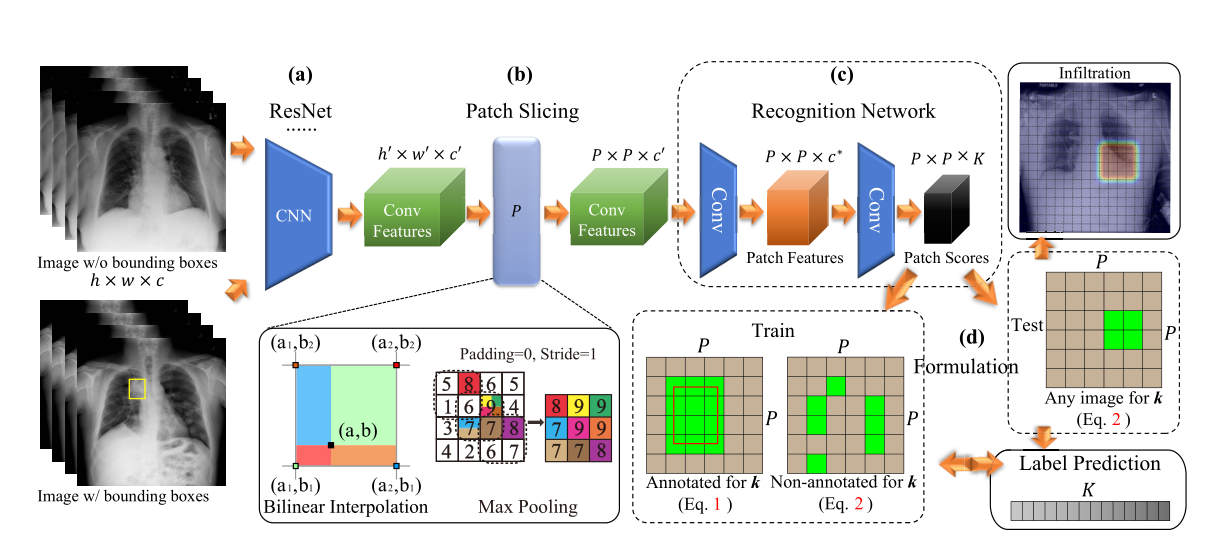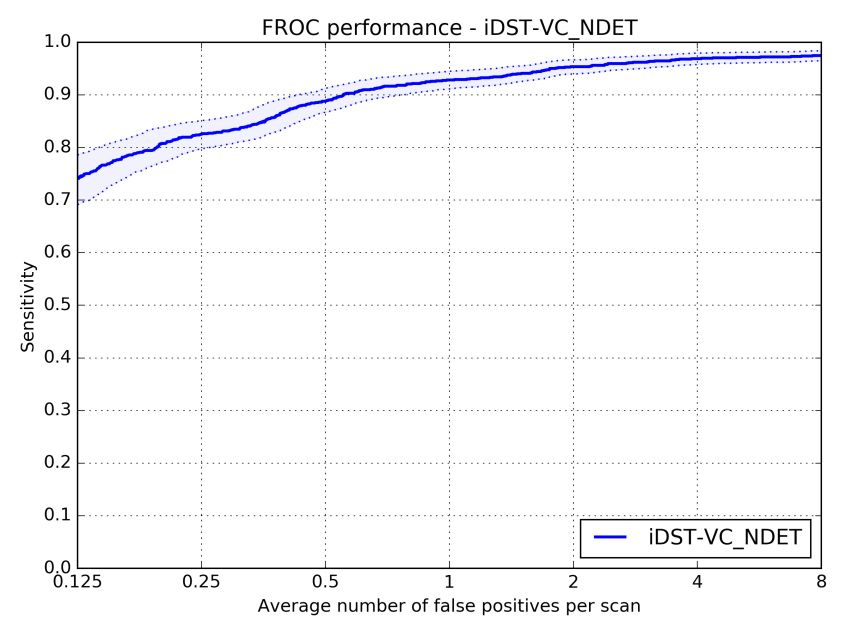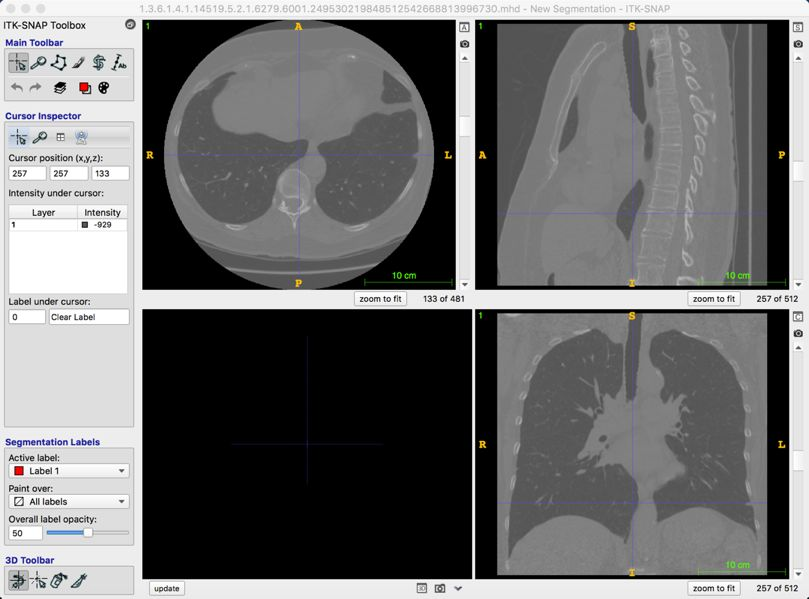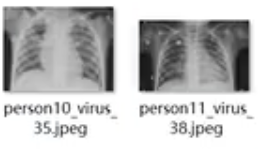# 面对疫情，AI能做什么### AI诊断医学影像，可能被用于疾病初筛

1.发热(≥38℃)；

2.具有肺炎或急性呼吸窘迫综合征的影像学特征；

3.发病早期白细胞总数降低或正常,或淋巴细胞分类计数减少；

4. 经抗生素规范治疗3~5天,病情无明显改善。而去年年末，国内顶级AI实验室腾讯优图，也将医疗 AI 深度学习预训练模型 MedicalNet （Github地址：https://github.com/Tencent/MedicalNet）正式对外开源，笔者看到这也是首个开源的能处理3D医学影像的深度学习框架。git clone https://github.com/Tencent/MedicalNet

然后到微云（https://share.weiyun.com/55sZyIx）下载相应模型，解压后既可进行训练。

python train.py --gpu_id 0 

# AI胸片图像辅助动手实践import keras
import pandas as pd
import numpy as np
import os
from tensorflow import set_random_seed
from keras.models import Sequential
from keras.layers import Dropout
from keras.layers import Dense, Flatten
from keras.layers import Conv2D, MaxPooling2D
from keras.layers import BatchNormalization
from keras.preprocessing.image import ImageDataGenerator

#定义文件路径
homepath='E:/ZhangLabDataset/'
#简单分类模型使用4层卷积，2层全连接，激活函数使用relu
np.random.seed(1)
set_random_seed(2)
model = Sequential()
input_shape=(128,128,1)))
gen = ImageDataGenerator(keras.utils.Sequence)
train_batches = gen.flow_from_directory(os.path.join(homepath,"train"),model.input_shape[1:3],color_mode="grayscale",shuffle=True,seed=1,batch_size=128)
valid_batches = gen.flow_from_directory(os.path.join(homepath,"val"), model.input_shape[1:3],color_mode="grayscale",shuffle=True,seed=1,batch_size=16)
test_batches = gen.flow_from_directory(os.path.join(homepath,"test"), model.input_shape[1:3], shuffle=False,color_mode="grayscale", batch_size=4)
model.fit_generator(train_batches,validation_data=valid_batches,epochs=4)
model.save('model.mdl')



# 最后是拉票环节©️2019 CSDN 皮肤主题: 技术黑板 设计师: CSDN官方博客